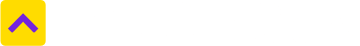# Convert Square Centimeter to Square Meter

One Square Centimeter is equal to 0.0001 Square Meter
Select State
Select from unit
Square Centimeter
Select to unit
0.0001
Square Meter

Square centimetre is a unit of area measurement, which is equal to a square measuring one centimeter on each side. Even though internationally the spelling square centimeter is used, the unit is also spelled as square centimeter, especially in the US. The abbreviations used for this measurement unit include cm2 and sq. cm. While the cm is an abbreviation for centimeter, the two represents squared. Sq. cm is ideal for measuring small areas and is primarily used for making measurements on drawings. As a centimeter is a metric unit of measurement, rules of the metric system must be applied to measure square centimeters.

1 sqcm is equal to:

100 square milimetre

0.15500031 square inch

0.00107639 square foot

0.00000002 acre

0.00000001 hectare

### What are the most common land measurement units in India?

Hectares, acres, square meters and square yards are some of the common land measurement units used across India. While Bigha and Marla are commonly used in the North, terms such as Cent, Guntha and Ground belong to the South. Depending upon the names of the units, their sizes also vary from State to State.

### How much is Square Meter in Square Centimeter terms?

One Square Meter is equal to 10000.0 Square Centimeter. Click here to convert

### How much is Square Centimeter in Square Meter terms?

One Square Centimeter is equal to 0.0001 Square Meter.

## Square Centimeter to other unit

Trusted by
Partners© 2012-21 Locon Solutions Pvt. Ltd.